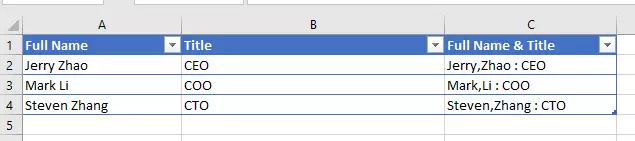# How To Add Comma Between Words In Excel

In this article, I will tell you how to add commas between words in Excel , how to add comma between first and last name in Excel, and how to add comma after first word in each cell.

## 1. How To Add Commas Between Words In Excel.

### 1.1 If The Words Are Saved In Separated Cells.

1. If you have a list of words in separate cells, you can use the CONCATENATE function to combine them with a comma in between each word.
2. For example, if you have the words ‘cat‘, ‘dog‘, and ‘bird‘ in cells A1, A2, and A3, respectively, you can use the following formula to combine them into one string separated by commas: =CONCATENATE(A1,”,”,A2,”,”,A3). The result would be ‘cat,dog,bird‘.

### 1.2 If The Words Are Saved In One Cell.

1. If all the words are in one cell, you can use the SUBSTITUTE function to replace spaces with commas.
2. For example, if you have the words ‘cat dog bird‘ in cell A1, you can use the following formula to replace the spaces with commas.
3. The formula is =SUBSTITUTE(A1,” “,”,”). The result would be ‘cat,dog,bird‘.

## 2. How To Add Comma Between First And Last Name In Excel.

1. In this case, we store user name ( First-Name & Last-Name) in one cell, the first name and the last name are separated by space.
2. You can use the SUBSTITUTE functions to add a comma between them also.
3. For example, if you have the user’s full name in cell A1, you can use the following formula to add a comma between the first and last name.
4. The formula is =SUBSTITUTE(A1,” “,”,”,1). The result would be ‘FirstName,LastName‘.

## 3. How To Add Comma After First Word In Each Cell In Excel.

1. First, input the below data in excel cells.
 1 A B C D 2 Jerry Zhao CEO 3 Mark Li COO 4 Steven Zhang CTO
2. Select the cell range A1:B3, then click the Home tab.
3. Click the Format as Table drop-down list, and select a table style.